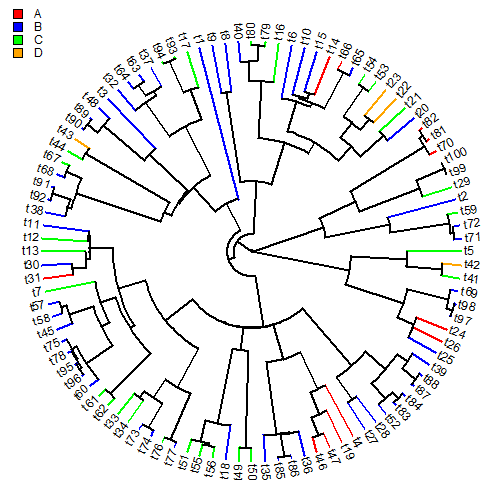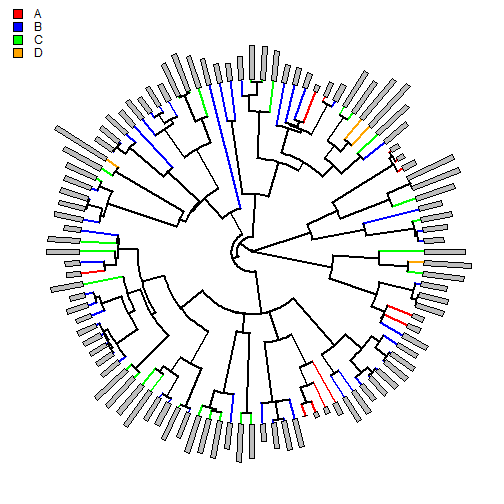## Saturday, October 11, 2014

### Colors terminal edges in plotTree.wBars by a discrete trait

A phytools user recently wrote in to ask if she could color the terminal edges of a phylogeny plotted using `plotTree.wBars` different colors depending on a discrete trait. Well, we already know that it's possible to plot a tree with a mapped discrete character. So to accomplish this, we just need to paint the terminal edges of the tree using the phytools function `paintSubTree`.

Here's a demo using simulated data:

``````library(phytools)
## let's simulate a tree & some data
tree<-pbtree(n=100,scale=1)
x<-fastBM(tree)
x<-x-min(x) ## set min to zero
th<-setNames(c(max(x)/4*1:3,Inf),LETTERS[1:4])
y<-sapply(x,threshState,th)
y
``````
``````##  t71  t72  t59   t2  t29  t99 t100  t70  t81  t82  t20  t21  t22  t23  t53
##  "B"  "B"  "C"  "B"  "C"  "D"  "D"  "A"  "A"  "A"  "B"  "C"  "D"  "D"  "C"
##  t54  t65  t66  t14  t15  t10   t6  t16  t79  t80  t40   t8   t9   t1  t17
##  "C"  "B"  "A"  "A"  "B"  "B"  "B"  "C"  "C"  "C"  "B"  "B"  "B"  "B"  "C"
##  t93  t94  t37  t63  t64  t32   t3  t48  t89  t90  t43  t44  t67  t68  t91
##  "C"  "C"  "B"  "B"  "B"  "B"  "B"  "B"  "B"  "B"  "D"  "C"  "C"  "B"  "B"
##  t92  t38  t11  t12  t13  t30  t31   t7  t57  t58  t45  t75  t78  t95  t96
##  "B"  "B"  "B"  "C"  "C"  "B"  "A"  "C"  "B"  "B"  "B"  "B"  "B"  "B"  "B"
##  t60  t61  t62  t33  t34  t73  t74  t76  t77  t51  t55  t56  t18  t49  t50
##  "B"  "C"  "C"  "C"  "C"  "B"  "B"  "C"  "B"  "C"  "C"  "C"  "B"  "C"  "C"
##  t35  t85  t86  t36  t46  t47  t19   t4  t27  t28  t52  t83  t84  t87  t88
##  "B"  "B"  "B"  "B"  "A"  "A"  "A"  "A"  "B"  "B"  "B"  "B"  "B"  "B"  "B"
##  t39  t25  t26  t24  t97  t98  t69  t41  t42   t5
##  "B"  "B"  "A"  "A"  "B"  "B"  "B"  "C"  "D"  "C"
``````

OK, so now we have data approximating the empirical data and we can attempt to generate our simulation. We will do this by first coloring all the terminal edges of the tree according to our discrete character, and then, having done that, we will run `plotTree.wBars` using the `method="plotSimmap"`.

``````## first color the tip edges
for(i in 1:length(y)) tree<-paintSubTree(tree,node=i,state=y[i],stem=TRUE)
colors<-setNames(c("black","red","blue","green","orange"),c(1,LETTERS[1:4]))
plotSimmap(tree,type="fan",colors=colors)
``````
``````## setEnv=TRUE for this type is experimental. please be patient with bugs
``````
``````add.simmap.legend(colors=colors[2:5],prompt=FALSE,x=0.95*par()\$usr,y=0.95*par()\$usr)
````````````## now plot with bars
plotTree.wBars(tree,x,method="plotSimmap",colors=colors,type="fan",scale=0.1)1.